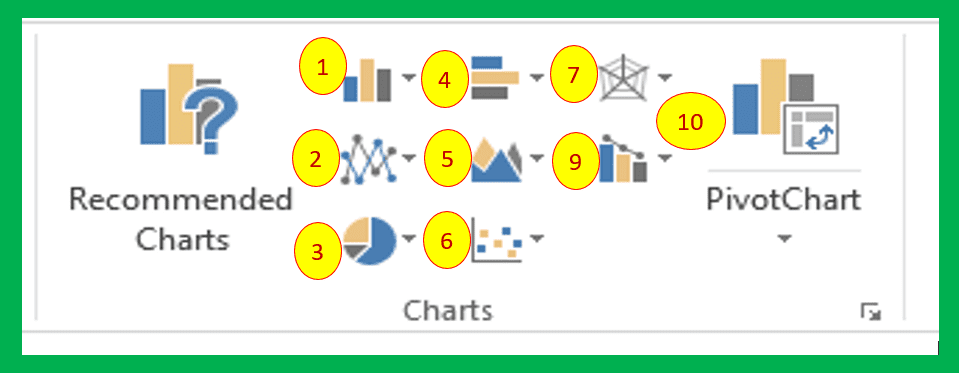Topics Covered

## Fundamental of charts in Excel

In the previous chapter, Writing excel formulas you learned how to write and use formulas in excel and preparing data or worksheets. In your worksheet, there are data of students’ marks, customer data, sales data, employees’ payroll data, or any other data. These data may useful for performing complex computations or analyses. But if you want to generate a report or represent similar data in pictorial form, you can use the charts in excel or graph in excel.
Charts are graphical or pictorial representation of data.

## Advantages of creating charts in excel

• Provides visualization
• Proper understanding
• The flow of data explanation
• Makes your data more attractive and presentable
• Increases User’s engagement
• Help see the relationship between data

## Types of charts in Excel | Excel Graph

Excel offers several types of charts. These types of charts you will see when you click on the Insert tab in MS-Excel from the charts group. Observe in the following screen:

This chart group provides the following chart types, as marked by the group with numbers.
1. 2D – 3D Column Chart
2. 2D – 3D Line Chart
3. 2D – 3D Pie and Doughnut Chart
4. 2D – 3D Bar Chart
5. 2D – 3D Area Chart
6. Scatter and Bubble Chart
7. Stock, Surface and Radar Chart
8. Combo Chart
9. Pivot Chart

### 1. Column Chart

The column chart is generally used to compare the data. It shows periodically changes in the data. In a cricket match, you might have seen this chart which displays the bowler’s bowling spell analysis or team score at 10 overs interval.  As you are aware that there will be two axes in the chart x-axis and y-axis. Now observe the following data:

Now I am going to represent this data in a column chart. The first option is a 2D chart which represents the data as a whole in different columns.

Observe both column charts and understand the difference between them.

### 2. Line Chart

Line charts are usually used to compare trends in data at equal intervals. It shows the changes over a period of time. It is useful for plotting the values when you have different trend values. In cricket matches, you might have seen these charts with a comparison of two team scores. Observe this data now:

So in the above data Score of each house is inserted for Term I and Term II. Now just I am creating 2D line chart in Excel for the same and displayed in the following screenshot.

Now observe the 3D line chart in Excel with the same data.

### 3. Bar Chart

Bar chart is reverse form of column chart. As in column chart categories are organized horizontally and values vertically, in bar chart categories are organized vertically and values horizontally. Observe the following screenshot:

The 3D bar chart is displayed in the following screenshot.

## 4. Scatter Chart

A Scatter chart is a little bit similar to the line chart that displays only dots plotted on the plot area. Observe the following screenshot.

## 5. Pie Chart

It is used to plot the values for a single data series. You can display the graphics for the entire year’s performance in slices.

Now observe the following screenshot:

## 6. Doughnut Chart

It is used to shows the relationships between different parts and makes one circle. It contains more than one data series. It shows one circle for each and one data series.

## 7. Area Chart

It is a filled colored version of a line chart. It fills a color between two lines. It highlights the size of the change in data over time.

In this chart, data will be plotted in concentric circles. Each data series is plotted along a separate axis from the center point to the outer ring. The line connects values from the series.

## Creating a chart in Excel | Creating a graph in Excel

Creating a chart in excel is quite simple and easy. Follow the following steps to create a chart in excel.
Step 2: Select the data which you want to plot on the chart.
Step 3: Click on insert → select desired chart type.

## Components of chart | Chart Elements

If you want to see all the chart elements then follow the following steps after creating the chart:
Step 1: Click on the chart.
Step 2: A + symbol will appear to the right side of your chart as displayed in the following screenshot.

Step 3: Click on the circled + button it will display drop-down with all chart elements as displayed in the following screenshot.

Step 4: Click on respective chart element to show/hide on the chart.

##### One thought on “Comprehensive note s Creating charts in Excel Class 7”
1. […] Use this link and find the answers (not provided): Creating charts in Excel […]